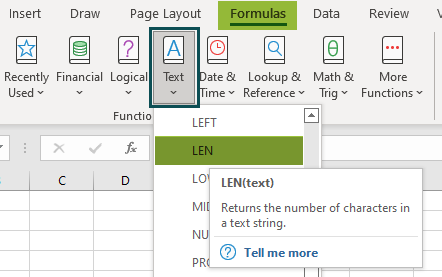# LEN Excel FunctionArticle byExcelMojo Team## What is LEN Function in Excel?

The LEN excel function counts the number of characters of a cell. Precisely, it counts letters, numbers, special characters, punctuation marks, and all kinds of spaces (leading, trailing, and between the words). The LEN returns only a numerical value as the output. It is categorized as a Text function and is available in almost all versions of Excel.

For example, in the following image, the formula “=LEN(A2)” counts the length of the string of cell A2.

The output of the LEN formula is 5. This implies that there are five characters in the string “apple.”

###### Key Takeaways
• The LEN function of Excel counts the characters of a text string, number or cell reference. It is a Text function of Excel.
• The syntax of the LEN function is “LEN(text).” The “text” argument is mandatory.
• The text strings supplied directly to the LEN function must be enclosed in double quotation marks.
• The TRIM function removes the extra spaces of a text string and retains only a single space between the words. The SUBSTITUTE function replaces one or more instances of a character (of a string) with the other.

### LEN() ExcelFormula

The formula of the LEN function in Excel is shown in the following image:

The LEN function accepts the following mandatory argument:

Text: This is the string whose characters are to be counted. It can be supplied as a direct text string (enclosed within double quotes), number or cell reference.

### How To Use The LEN Function In Excel?

The LEN function of Excel can be used in either of the following ways:

1. Access the LEN function from the Excel ribbon

1. Choose an empty cell that will contain the output.

2. Go to the Formulas tab of Excel.

3. Expand the “text” drop-down from the “function library” group.

4. Choose “LEN” from the list of functions. A window called “function arguments” opens.

5. Enter the single argument in the “text” box and click “OK.”

2. Enter the LEN function in the worksheet manually

1. Select an empty cell for the output.
2. Type “=LEN(” in the selected cell. Alternatively, type “=L” and double-click the LEN function from the list of suggestions shown by Excel.
3. Enter the argument as a cell reference or a direct value.
4. Close the parenthesis and press the “Enter” key.

The following image shows a phrase (or string) in cell A2. Count the length of this string by using the LEN function of Excel.

The steps to find the length of the given string are listed as follows:

Step 1: Enter the following LEN formula (without the double quotation marks) in cell B2.

“=LEN(A2)”

After opening the LEN function, one can either select cell A2 or type the cell reference manually.

Step 2: Press the “Enter” key. The output in cell B2 is 25, as shown in the following image. So, this count includes 21 characters and 4 spaces between the words.

### Examples

#### Example #1 – Calculate The Character Count When The Inputs Are Numerical And Textual

The following image shows some numerical and textual values in column A. Count the number of characters in each cell of range A2:A7. Use the LEN function of Excel.

The steps to perform the given task are listed as follows:

Step 1: Enter the following LEN formula in cell B2.

“=LEN(A2)”

To supply the reference, either type it or select cell A2.

Step 2: Press the “Enter” key. The output in cell B2 is 4, as shown in the following image.

Step 3: Drag the formula of cell B2 till cell B7. For dragging, use the fill handle at the bottom-right corner of cell B2. The outputs are shown in the following image.

The LEN function has returned the character count of each cell of the range A2:A7. It has counted both numbers and text values. It has returned 5 for the date of cell A6. This is because the date 29-03-2022 is represented by the serial number 44649, which consists of five digits.

Hence, the LEN function has not counted the date in the displayed format (dd-mm-yyyy).

Note: Excel stores dates as serial numbers starting from number 1. The number 1 represents the date January 1, 1900.

#### Example #2–Calculate The Word Count When The Input Is a Statement

The succeeding image shows a statement (or string) in cell A2. Count the number of words in this string by using the LEN, TRIM, and SUBSTITUTE functions of Excel. Explain the formula thus used.

TRIM function in Excel

The TRIM function in Excel removes the extra spaces of a text string, whether leading, trailing or in-between the words. However, the function retains a single space between the words of the output.

The syntax of the TRIM function is stated as follows:

“TRIM(text)”

The “text” is the cell whose extra spaces are to be removed. A direct text string can also be supplied to the TRIM function, provided it is enclosed in double quotation marks. The “text” argument is mandatory.

SUBSTITUTE function in Excel

The SUBSTITUTE function in Excel replaces one character of a text string with another. It can also replace multiple occurrences of multiple characters.

The syntax of the SUBSTITUTE function is stated as follows:

“SUBSTITUTE(text,old_text,new_text,[instance_num])”

• Text: This is the string within which characters are to be substituted.
• Old_text: This is the character that must be replaced.
• New_text: This is the character with which the “old_text” must be replaced.
• Instance_num: This decides which occurrence of the “old_text” will be replaced with the “new_text.” For example, if “instance_num” is 1, the first occurrence of the “old_text” is replaced with the “new_text.”

The arguments “text,” “old_text,” and “new_text” are mandatory, while “instance_num” is optional. If the “instance_num” argument is omitted, every occurrence of the “old_text” is replaced with the “new_text.”

The steps to count the number of words in cell A2 are listed as follows:

Step 1: Enter the following formula in cell B2.

“=LEN(TRIM(A2))-LEN(SUBSTITUTE(A2,” “,””))+1”

Step 2: Press the “Enter” key. The output in cell B2 is 11, as shown in the following image.

Explanation of the formula: The LEN, TRIM, and SUBSTITUTE formula of step 1 is explained as follows:

• The SUBSTITUTE function replaces all spaces (“ ”) of cell A2 with empty strings (“”). So, the space is the “old_text” argument and the empty string is the “new_text” argument. The SUBSTITUTE function returns the statement without spaces (Abirdinthehandisworthtwointhebush.)
• The output of the SUBSTITUTE function becomes the input of the nested LEN function. So, this inner LEN returns the character count of the statement (excluding the spaces and including the period), which is 34.
• The TRIM function removes the extra spaces of cell A2. It returns the statement (or string), “A bird in the hand is worth two in the bush.”
• The output of the TRIM function becomes the input of the outer LEN function. The outer LEN returns 44, which is the total length of the string. The length of the string without spaces (34) is subtracted from the total length of the string (44). This returns the number of spaces in the string, which is 10.
• The number 1 is added to the number of spaces of the string. This is because the number of words in the statement is equal to the number of spaces (10) plus one. So, 44-34+1=11.

Therefore, the statement of cell A2 contains 11 words.

#### Example #3–Calculate The Character Count When The Input Is a Blank Cell Reference

The following image shows cell A2 as a blank cell. Supply this single blank cell to the LEN function and observe the outcome.

The steps to find the length by supplying a blank cell are listed as follows:

Step 1: Enter the following formula in cell B2. Exclude the beginning and ending double quotation marks while entering this formula in Excel.

“=LEN(A2)”

Step 2: Press the “Enter” key. The output is 0. This is shown in cell B2 of the following image.

Hence, since cell A2 does not contain any character, the LEN function returns 0. Had there been a single space in this cell, the LEN would have returned 1.

### Important Things To Note

The important points related to the LEN function of Excel are listed as follows:

• It counts the number of characters of a cell.
• It returns only numeric values and can also be used in Excel VBA.
• It returns the word count of strings when used with the TRIM and SUBSTITUTE functions of Excel.
• It returns 0 if a blank cell is supplied to it.

1. Define the LEN function of Excel.

The LEN function returns the length of the supplied string. This function can be supplied cell references or range references containing text strings, numbers, spaces, and special characters. Text strings supplied directly must be enclosed within double quotation marks.

The syntax of the LEN function of Excel is stated as follows:

“LEN(text)”

The “text” argument is mandatory.

Note: For the working of the LEN function, refer to the examples in this article.

2. How to use the LEN function in Excel?

Let us use the LEN function to count the characters of each cell of the range A2:A4. The values are shown in the following image.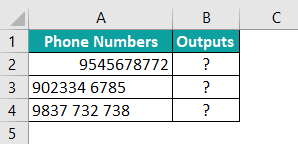The steps for using the LEN function in Excel are listed as follows:

Step 1: Enter the following LEN formula in cell B2. The beginning and ending double quotation marks need not be entered in Excel.
“=LEN(A2)”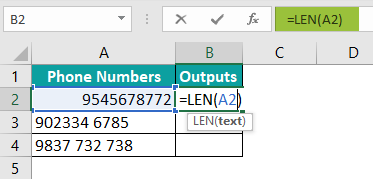Step 2: Press the “Enter” key. The output is 10, as shown in the following image.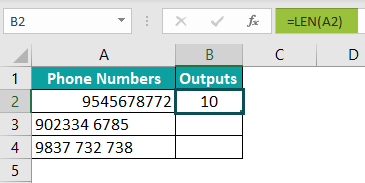Step 3: Drag the formula of cell B2 till cell B4 by using the fill handle. The LEN function has returned the count of numbers of cells A2, A3, and A4 in the following image.

Notice that the numbers in each of the three cells are 10. However, cells A3 and A4 contain spaces too. The former contains one space, while the latter contains two space characters. These spaces are included in the output of the LEN function.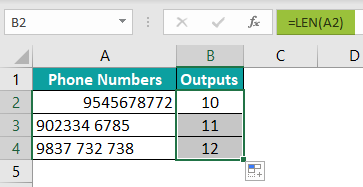3. Where is the LEN function in Excel?

The steps to access the LEN function of Excel are listed as follows:

a. Click the “text” drop-down from the “function library” group of the Formulas tab.
b. Select the “LEN” function.
c. Specify the single argument in the “function arguments” window.
d. Click “OK” to find the character count.

The selections of steps “a” and “b” are shown in the following image.